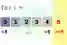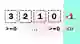# Java For Loop Examples

Iterate over numeric ranges and collections with the for-loop. Compare different loops.

For. Most runtime in programs is spent in loops. The for-loop iterates over numbers. It is commonly used. It is ideal for processing known ranges.Keyword notes. In Java, no foreach keyword is used. Instead we use the for-keyword to iterate over each element in a collection. We do not need an index to do this.

Example. This program uses a simple for-loop. It declares the iteration variable "i" inside the condition of the loop. It starts at 0, and continues until it is equal to 5.
Part 1: The first part of the for-loop condition is where the loop iteration variable is declared and initialized.
Part 2: This is the terminating condition. In this loop, we terminate when the variable reaches 5.
Part 3: The third and final part of the for-loop is the iteration statement. This is applied after each pass through the loop.Java program that uses for-loop public class Program { public static void main(String[] args) { // Part 1: start at 0. // Part 2: continue until 5 is reached. // Part 3: increment by 1. for (int i = 0; i < 5; i++) { System.out.println(i); } } } Output 0 1 2 3 4

Decrement loop. Often we use decrementing for-loops to iterate backwards through a series of numbers. The >= operator means we include zero in the loop body.Java program that decrements in for-loop public class Program { public static void main(String[] args) { // Loop from 3 to 0, decrementing. for (int i = 3; i >= 0; i--) { System.out.println(i); } } } Output 3 2 1 0

For each. This is a simple syntax form. If we loop over a collection, we use a colon, not an index variable. This enumerates each element in the collection (array, ArrayList).Array
Java program that uses for, alternate syntax public class Program { public static void main(String[] args) { String[] values = new String; values = "Dot"; values = "Net"; values = "Perls"; for (String value : values) { System.out.println(value); } } } Output Dot Net Perls

Break. A for-loop can be stopped at any time, based on any condition. We use the "break" statement. It takes no arguments and terminates the nearest enclosing loop.Break
Tip: More complex logic is needed to fully break out of a nested loop. A flag boolean, or the use of methods, is needed.
However: This for-loop example scans each element in the values array. It stops (breaks) when a negative one element is found.
Java program that uses break public class Program { public static void main(String[] args) { int[] values = { 1, 2, 3, -1 }; // ... Loop over array indexes, but break on negative one. for (int i = 0; i < values.length; i++) { if (values[i] == -1) { break; } System.out.println(values[i]); } } } Output 1 2 3

Continue. This keyword stops the current loop iteration and moves to the next one. Further statements are not executed. In a loop with an iteration condition, the next iteration begins.Continue
Caution: A continue statement can sometimes lead to an infinite loop. Be sure the iteration variable is incremented.
Here: We use "continue" to skip over Strings in an array that start with the letter "b." We filter Strings.
Java program that uses continue public class Program { public static void main(String[] args) { String[] values = { "cat", "bear", "dog", "bird" }; // Loop over all Strings. for (String value : values) { // Skip Strings starting with letter b. if (value.startsWith("b")) { continue; } System.out.println(value); } } } Output cat dog

Nested for-loops. All kinds of loops can be nested. When we use a break or continue statement with a nested loop, only the innermost loop is affected.
However: A return statement will exit all loops in the current method. Sometimes flag variables of boolean type are needed.
Java program that uses nested for-loops public class Program { public static void main(String[] args) { // Use nested for-loops. for (int i = 0; i < 3; i++) { for (int y = 0; y < 3; y++) { System.out.println(i + "," + y); } } } } Output 0,0 0,1 0,2 1,0 1,1 1,2 2,0 2,1 2,2

Reuse iteration variable. Sometimes an iteration variable needs to be reused outside of a for-loop. We can use any local variable in a new for-loop.
And: The local variable, like x in this program, remains reachable after the loop itself terminates.
Java program that reuses iteration variable public class Program { public static void main(String[] args) { int x = 0; // Parts of the for-loop can be omitted. // ... Here we use no variable declaration in the for-statement. for (; x < 3; x++) { System.out.println(x); } System.out.println("x is still reachable!"); System.out.println(x); } } Output 0 1 2 x is still reachable! 3

Method, for-each. A method can be called in the for-loop. This method is evaluated once and then the results of it are accessed in the loop iteration variable.
Here: This program shows that the method getElements is only called once. It is not called three times.
So: When calling a variable or method in a for-each loop, we can see that the result is cached in a local and not evaluated more than once.
Java program that uses method, for-each loop public class Program { static int count; static int[] getElements() { // Set array elements based on a static field. int[] array = new int; array = count++; array = count++; array = count++; return array; } public static void main(String[] args) { // The method is called once and not many times in the for-loop. for (int value : getElements()) { System.out.println(value); } } } Output 0 1 2

Overflow behavior. Loops in Java can wrap around. This can happen in loops where we increment or decrement. The int type overflows and becomes negative, then reaches the target.
Caution: This mistake can cause a serious performance problem. Be sure to validate your looping logic. A for-each loop also helps.
Java program that has loop overflow error public class Program { public static void main(String[] args) { long iterations = 0; // Count iterations from 100 to 200 decrementing. for (int u = 100; u <= 200; u--) { iterations++; } System.out.println("Iterations from 100 to 200: " + iterations); } } Output Iterations from 100 to 200: 2147483749

String loop. This program uses a for-loop over a String. We start at index 0 and process until the length() is reached. With charAt we get each character at an index.Strings
Java program that uses for-loop, string public class Program { public static void main(String[] args) { String value = "art"; // Loop from 0 to length() of the string. for (int i = 0; i < value.length(); i++) { // Get letters with charAt method. char letter = value.charAt(i); System.out.println(letter); } } } Output a r t

Benchmark, loop jamming. In loop jamming many loops are combined into one. Consider this program—three arrays, all of the same length, must be looped over.
Version 1: The first part loops over the three arrays separately and sums their elements.
Version 2: The second part loops over the arrays in a single, jammed loop. This version is faster.
Result: The single loop is nearly twice as fast as the three loops put together. And it has the same result on every iteration.
Java program that applies loop jamming public class Program { public static void main(String[] args) { int[] array1 = { 10, 20, 30 }; int[] array2 = { 20, 10, 30 }; int[] array3 = { 40, 40, 10 }; long t1 = System.currentTimeMillis(); // Version 1: loop over each array separately. for (int i = 0; i < 10000000; i++) { int sum = 0; for (int x = 0; x < array1.length; x++) { sum += array1[x]; } for (int x = 0; x < array2.length; x++) { sum += array2[x]; } for (int x = 0; x < array3.length; x++) { sum += array3[x]; } if (sum != 210) { System.out.println(false); } } long t2 = System.currentTimeMillis(); // Version 2: jam loops together. for (int i = 0; i < 10000000; i++) { int sum = 0; for (int x = 0; x < array1.length; x++) { sum += array1[x]; sum += array2[x]; sum += array3[x]; } if (sum != 210) { System.out.println(false); } } long t3 = System.currentTimeMillis(); // ... Times. System.out.println(t2 - t1); System.out.println(t3 - t2); } } Output 109 ms, 3 for-loops 48 ms, 1 for-loop (jammed)

Benchmark, loop unrolling. Sometimes loops are unnecessary. In loop unrolling, we change a loop to a list of statements. We can loop over groups of statements at once.
Version 1: In this version of the code, we have a nested inner loop—this is the loop that is not optimized with unrolling.
Version 2: In this code we simply remove the entire loop and place the statements that need to be executed.
Result: This optimization can improve, or reduce, performance. Here, the unrolled statements are faster.
Java program that unrolls loops public class Program { public static void main(String[] args) { int[] array1 = new int; long t1 = System.currentTimeMillis(); // Version 1: assign elements in a loop. for (int i = 0; i < 10000000; i++) { for (int x = 0; x < array1.length; x++) { array1[x] = x; } } long t2 = System.currentTimeMillis(); // Version 2: unroll the loop and use a list of statements. for (int i = 0; i < 10000000; i++) { array1 = 0; array1 = 1; array1 = 2; array1 = 3; array1 = 4; } long t3 = System.currentTimeMillis(); // ... Times. System.out.println(t2 - t1); System.out.println(t3 - t2); } } Output 56 ms, for-loop 17 ms, unrolled statements

While. A for-loop is best when the starting and ending numbers are known. If the end index is unknown, consider a while-loop. Use a break when the end is reached.While

ArrayList. A for-loop can be used on an ArrayList. We can iterate through the indexes, bounded by size(), or directly through the elements with simpler syntax.ArrayList, For

A review. Loops are key to optimizations. Often we eliminate steps from the loop body by changing the loop declaration. Loops affect control flow, causing it to repeat.

Home
© 2007-2020 Sam Allen. Every person is special and unique. Send bug reports to info@dotnetperls.com.